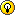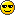SB Physics Help Room Sucks!
Would you like to react to this message? Create an account in a few clicks or log in to continue.

# chapter 27.1##chapter 27.1

anyone understand how to do question # 3?
i've been trying # 3 for a while and im having no luck

phyfrk
Guest

##Question 3

The answer to question 3 is
2.3*10^17.
The radius is constant and we use the equation
Density=Mass/Volume
So..
Density=(1.66e-27)/((4pi/3)*(1.2e-15)^3)
Gives us
2.3e17
This is constant no matter what Atomic Number is given in your problem.

Guest 24
Guest

##Question #1

Does anyone know how to do #1, i tried doing it and it isnt working.

Guest001
Guest

### 3

thanks guest 24 for explaining # 3

phyfrk
Guest

##question 2

I need help with number 2 does anyone have any idea?

cute611
Guest

###2

Is something wrong with MapleTA acceptiing my answer?
Atomic mass unit = 221 (given)
(221)^1/3 * 1.2*10^-15 = 7.25513*10^-15
I don't know what I am doing wrong and Allen does not have the blog updated.

super mo
Guest

###2

Stupid mistake - I got itsuper mo
Guest

##Question 1

Question 1:

A neutron (its mass=1.67x10-27kg can be approximated as 1 u) makes an elastic head-on collision with a nucleus which is at rest and has the atomic weight 4.443 u. Calculate the velocity of the recoiling nucleus as a multiple of the velocity of the incident neutron (sheet 4). Indicate with a positive (negative) sign whether the neutron and proton masses are about equal (very different from each other).

Use the equation v_2 = ((2*m_1)/(m_1+m_2))*v_1

Let the neutron mass = m_1
Nucleus mass = m_2
Plug into the equation and you end up with v_2 = ####*v_1
That number is your answer because they ask for the velocity of the nucleus as a multiple of the velocity

KT
Guest

##Question 2

Question 2:

The Atomic Mass Number of a nucleus is 32. Calculate the nuclear radius in fm (sheet. Indicate with a positive (negative) sign whether the expression on sheet 8 implies that the nucleons compress or dip into each other (are considered incompressible and impenetrable).

Let A = 32
R_0 (constant) = 1.2E-15

Use the formula r = R_0*A^(1/3)
You will get something like r=#.###E-15 m
this answer is in meters but they want it in fm
To convert to fm, know that 10^-15 meters = 1fm, so just divide your answer by 10^-15 and you will get #.###

KT
Guest

##Question 4

Question 4:

The answer is just the number given in the problem. & it is negative.

KT
Guest

##what is v_1 in #1?

In the formula provided for question 1, what does v_1 =?
And, we're solving for v_2, right?

question
Guest

##Re: chapter 27.1

For Question 1, v_1 is equal to the incident velocity, and yes youre solving for v_2. The question asks you to solve for v_2 as a multiple of v_1, so thats why you end up with v_2 = number*v_1

KT
Guest

##Re: chapter 27.1

can someone help me with #1
I can't figure it out
what do u multiply by at the end ??

helpp
Guest

##1

I need help with 1 too

snowman
Guest

###1

For # 1 you just have to convert every thing in u to kg... and then plug in the formula given above. you should get the asnwer

ps
Guest

##Question 3

I'm confused with Question 3 . Why is A=1? how come the radius is just the value of R_0 ?

an0n
Guest

##Re: chapter 27.1